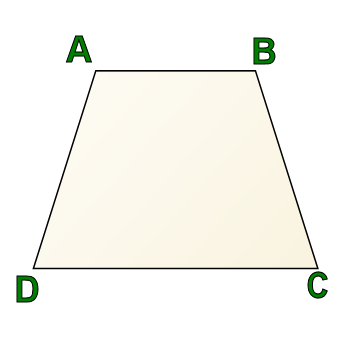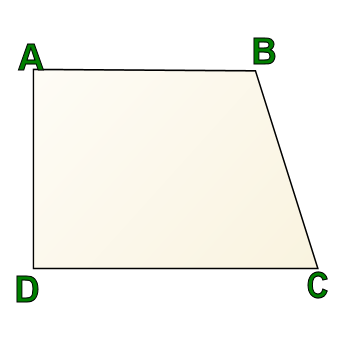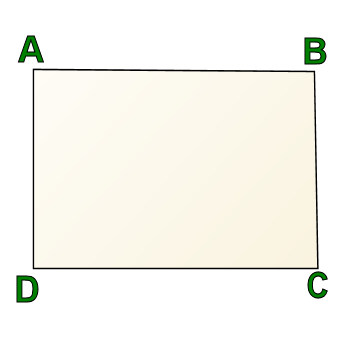Games
Problems
Go Pro!

Sixth grader Abdulla from U.A.E. asks the following question: "Are there perpendicular sides in trapezoid?"

The answer to that question, Abdulla, is "sometimes yes, sometimes no."

When people say the word "trapezoid," the image that automatically conjures up for most people looks like this:This is a trapezoid, and it is often described as a triangle with the top lopped off, or as a "dog food dish."

In this particular trapezoid, there are no perpendicular sides. A and B are both obtuse angles in this diagram, and C and D are both acute angles.

However, not all trapezoids look like this. Let's talk for a moment about the definition of a trapezoid.

Interestingly, the definition of "trapezoid" will vary depending on the part of the world in which you live, and in some parts of the world, the word "trapezium" is used for what I refer to as a trapezoid. I'm going to show you two definitions of trapezoid, and you'll need to check with your teacher/textbook to find out which way of defining a trapezoid is used in your part of the world.

Definition A: A trapezoid is any quadrilateral with exactly one pair of parallel sides.

Definition B: A trapezoid is any quadrilateral with at least one pair of parallel sides.Regardless of which definition you use, the following is a trapezoid:

AB is parallel to CD still, so it meets definition B, and since AD is not parallel to BC, it also meets definition A.

This trapezoid is called a "right trapezoid." Why? Because always true of trapezoids (regardless of your definition): Right angles come in pairs in a trapezoid.

Why? BecauseLet's take this one step further, though. Here's another figure. In this one, you have four right angles. That makes this a rectangle.

But is this figure also a trapezoid? It depends on whether you're using definition A or definition B.

If you're using definition A, it absolutely is not a trapezoid, because it has two pairs of parallel sides.

However, if you're using definition B, having two pairs of parallel sides is okay.

So if you're using definition B, then a trapezoid could be made up entirely of right angles, and a rectangle would be a special kind of trapezoid.

Otherwise, if you're using definition A, the most a trapezoid could have is two right angles, and therefore, two perpendicular sides.

Professor PuzzlerLike us on Facebook to get updates about new resources